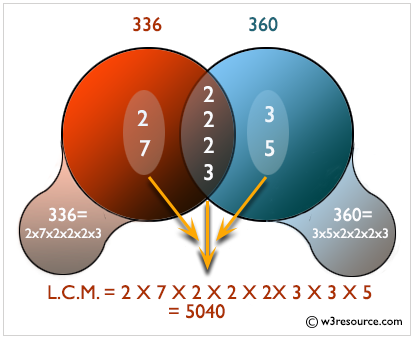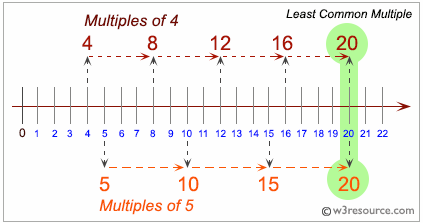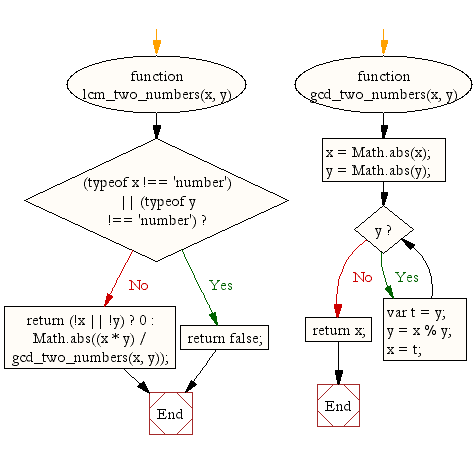# JavaScript: Least common multiple (LCM) of two numbers

## JavaScript Math: Exercise-10 with Solution

Write a JavaScript function to get the least common multiple (LCM) of two numbers.
Note :
According to Wikipedia - A common multiple is a number that is a multiple of two or more integers. The common multiples of 3 and 4 are 0, 12, 24, .... The least common multiple (LCM) of two numbers is the smallest number (not zero) that is a multiple of both.

Test Data:
console.log(lcm_two_numbers(3,15));
console.log(lcm_two_numbers(10,15));
Output :
15
30

Explanation of L.C.M.:Pictorial Presentation of L.C.M. of two numbers:Sample Solution:-

HTML Code:

``````<!DOCTYPE html>
<html>
<meta charset="utf-8">
<title>LCM of two numbers</title>
<body>
</body>
</html>
```
```

JavaScript Code:

``````function lcm_two_numbers(x, y) {
if ((typeof x !== 'number') || (typeof y !== 'number'))
return false;
return (!x || !y) ? 0 : Math.abs((x * y) / gcd_two_numbers(x, y));
}

function gcd_two_numbers(x, y) {
x = Math.abs(x);
y = Math.abs(y);
while(y) {
var t = y;
y = x % y;
x = t;
}
return x;
}
console.log(lcm_two_numbers(3,15));
console.log(lcm_two_numbers(10,15));
```
```

Sample Output:

```15
30
```

Flowchart:Live Demo:

See the Pen javascript-math-exercise-10 by w3resource (@w3resource) on CodePen.

Improve this sample solution and post your code through Disqus

What is the difficulty level of this exercise?

Test your Programming skills with w3resource's quiz.

﻿